# Jaccard-Index versus Accuracy#

Depending on the use-case some metrics are sub-optimal for determining segmentation quality. We demonstrate this by comparing segmentation results on differently cropped images.

from skimage.data import human_mitosis
from the_segmentation_game import metrics
import napari_segment_blobs_and_things_with_membranes as nsbatwm
import stackview


We use the human_mitosis example dataset from scikit-image.

image = human_mitosis()[95:165, 384:454]

stackview.insight(image)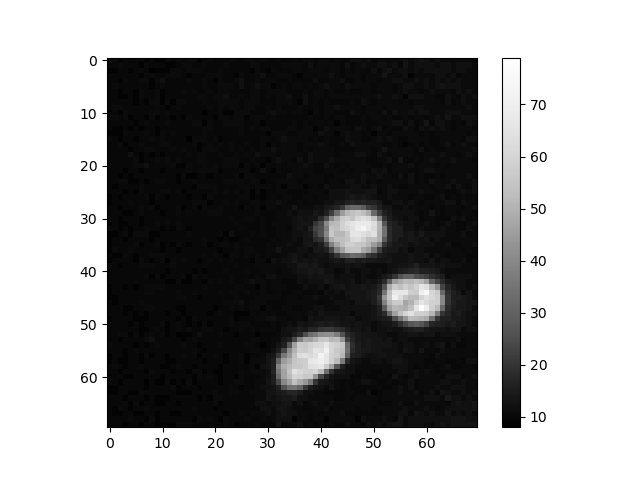shape (70, 70) dtype uint8 size 4.8 kB min 8 max 79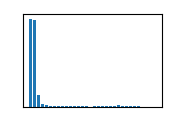Let’s assume this is a reference annotation performed by an expert.

reference_labels = nsbatwm.voronoi_otsu_labeling(image)
reference_labels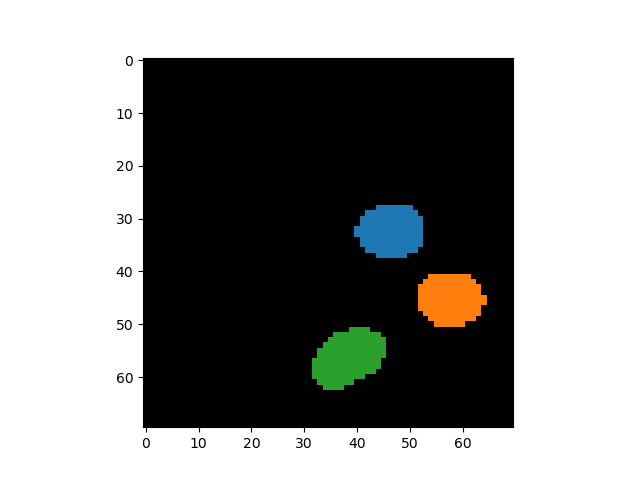nsbatwm made image
 shape (70, 70) dtype int32 size 19.1 kB min 0 max 3

Furthermore, this create a segmentation result we would like to determine the quality of.

test_labels = nsbatwm.gauss_otsu_labeling(image, outline_sigma=3)

test_labels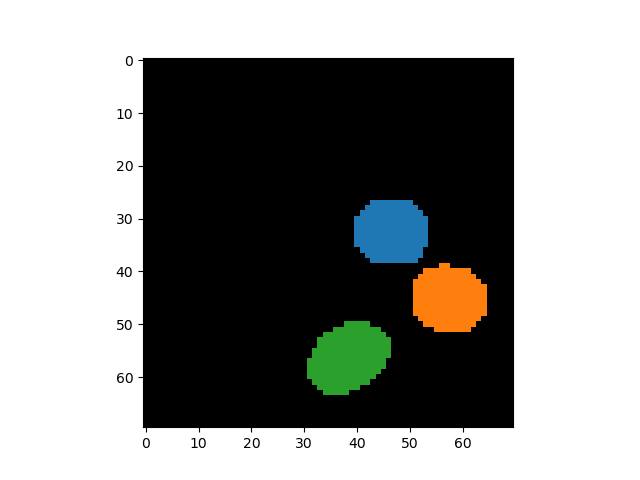nsbatwm made image
 shape (70, 70) dtype int32 size 19.1 kB min 0 max 3

## Quality measurement#

There are plenty of quality metrics for measuring how well the two label images fit to each other. In the following we use accuracy and jaccard index as implemented in The Segmentation Game, a napari-plugin for measuring quality metrics of segmentation results.

metrics.roc_accuracy_binary(reference_labels, test_labels)

0.9744898

metrics.jaccard_index_sparse(reference_labels, test_labels)

0.7274754206261056


We will now apply the same metrics to the label image again, but crop the label image by removing some of the zero-value pixels in the top and left of the label image.

metrics.roc_accuracy_binary(reference_labels[20:,20:], test_labels[20:,20:])

0.95

metrics.jaccard_index_sparse(reference_labels[20:,20:], test_labels[20:,20:])

0.7274754206261056


As you can see, the accuracy metric changes, while the Jaccard Index does not. Obviously the accuracy metric depends on the amount of zero-value pixels in the label image. We just visualize the cropped images:

reference_labels[20:,20:]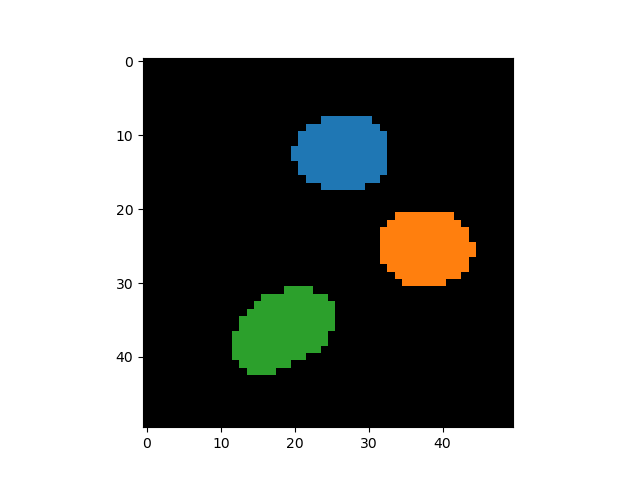nsbatwm made made image
 shape (50, 50) dtype int32 size 9.8 kB min 0 max 3
test_labels[20:,20:]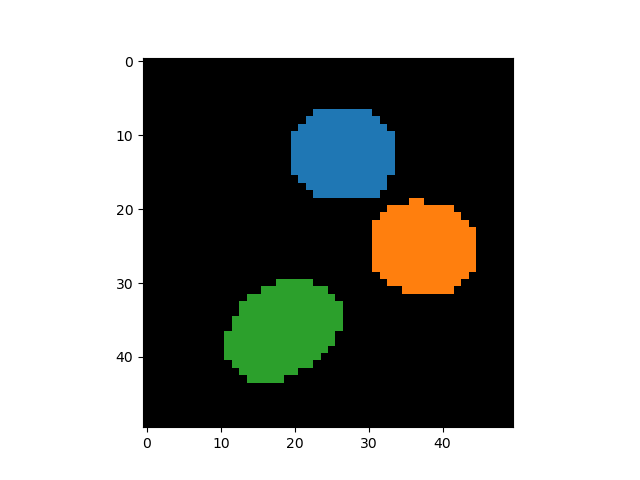nsbatwm made made image
 shape (50, 50) dtype int32 size 9.8 kB min 0 max 3

## Explanation#

When comparing the equations of accuracy $$A$$ and Jaccard index $$J$$, it is obvious that both do the same kind-of, but only accuracy includes the number of zero-value pixels in both label images. These pixels are the true-negatives $$TN$$.

$A =\frac{TP + TN}{FN + FP + TP + TN}$
$J =\frac{TP}{FN + FP + TP}$